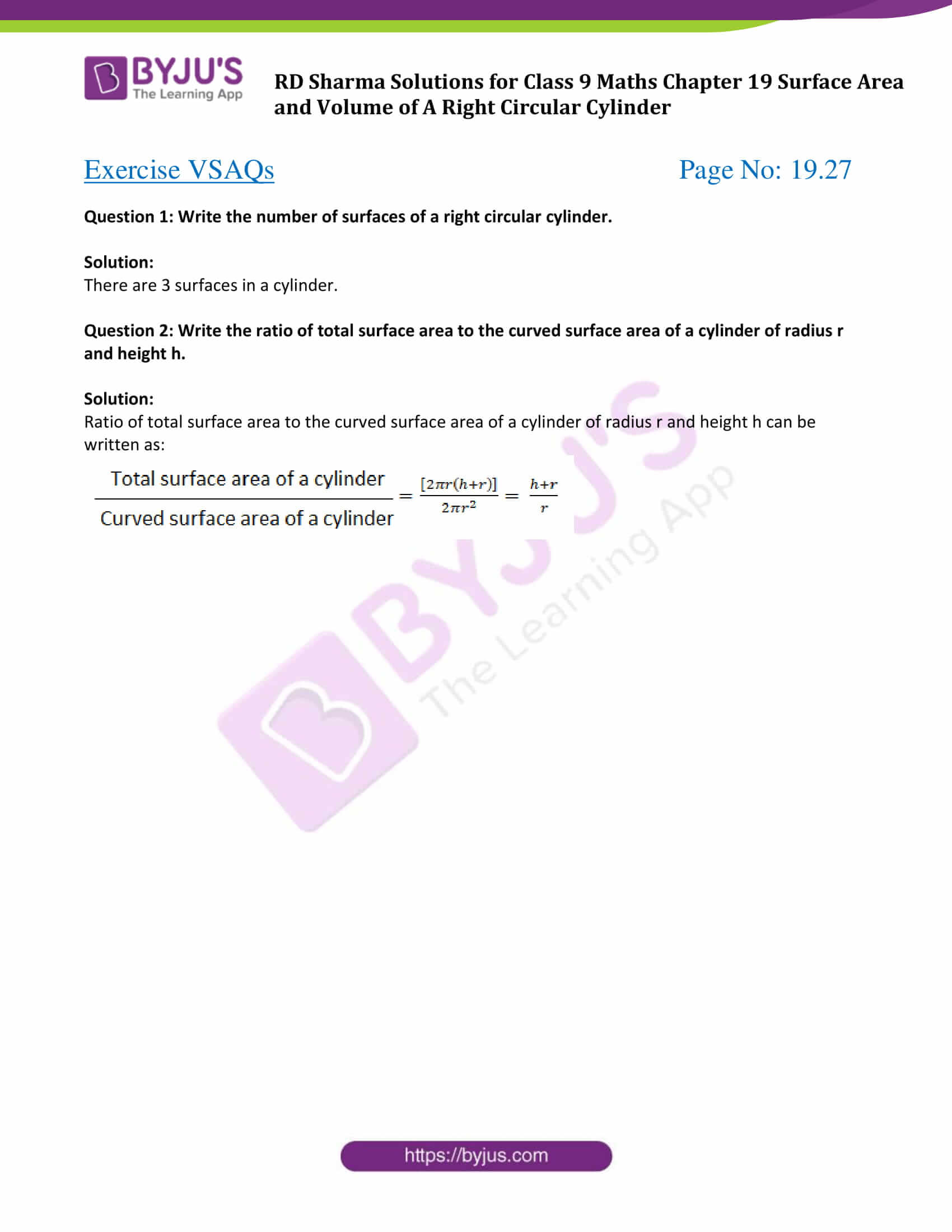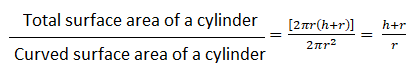# RD Sharma Solutions for Class 9 Maths Chapter 19 Surface Area and Volume of A Right Circular Cylinder Exercise VSAQs

Class 9 Chapter 19 – Surface Area and Volume of A Right Circular Cylinder Exercise VSAQs solutions are provided here. These solutions will help in constructive preparation and learning. The RD sharma class 9 PDF can be accessed here and students can also download it for future reference. Students can also check out RD Sharma solutions to practice other class 9 Maths topics. Students can find new ways to solve problems by practicing this study material.

## Download PDF of RD Sharma Solutions for Class 9 Maths Chapter 19 Surface Area and Volume of A Right Circular Cylinder Exercise VSAQs### Access Answers to Maths RD Sharma Solutions for Class 9 Chapter 19 Surface Area and Volume of A Right Circular Cylinder Exercise VSAQs Page number 19.27

Question 1: Write the number of surfaces of a right circular cylinder.

Solution:

There are 3 surfaces in a cylinder.

Question 2: Write the ratio of total surface area to the curved surface area of a cylinder of radius r and height h.

Solution:

Ratio of total surface area to the curved surface area of a cylinder of radius r and height h can be written as:## RD Sharma Solutions for Class 9 Maths Chapter 19 Surface Area and Volume of A Right Circular Cylinder Exercise VSAQs

RD Sharma Solutions Class 9 Maths Chapter 19 Surface Area and Volume of A Right Circular Cylinder Exercise VSAQs is based on calculating volume and surface area of geometric figure, cylinder. Students can download RD sharma Class 9 pdf to learn about right circular cylinder and learn how to find the surface area and volumes of this figure with the help of various practice problems.